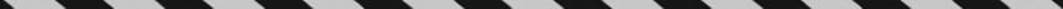﻿ ﻿PERCENT PROBLEMS - Word Problems - ALGEBRA - SAT SUBJECT TEST MATH LEVEL 1

## ALGEBRA## CHAPTER 7Word Problems### PERCENT PROBLEMS

If you have any difficulty with problems involving percents, including percent increase and percent decrease, review the material on percents in Chapter 3.

EXAMPLE 4: There are twice as many girls as boys in a biology class. If 30% of the girls and 45% of the boys have completed a lab, what percent of the students in the class have not yet completed the lab?

If x represents the number of boys in the class, then 2x represents the number of girls in the class. Then of the 3x students in the class, the number of students who have completed the lab isThe fraction of students who have completed the lab isSo 65% of the students have not yet completed the lab.

﻿